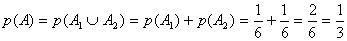# Translations of Encyclopedia about Mathematics

## Probability

If one were to ask someone who is intensively involved in probability what this term means, they might receive a response like: “An event which has a high probability occurs often and an event which has a low probability occurs less often.” Or: “If the probability that event A will occur is greater than that of event B, then event A will occur more often than event B.” In many cases, we would prefer more exact information, such as “how much” it would be probable that one event will occur and another will not. The modern mathematical theory of probabilities, founded by Russian mathematician Kolmogorow, has the sufficient tools to accomplish this and is the theoretical foundation and a part of .

Random Event

When performing many experiments, one of many events can occur. Although this means that various results may occur, prior to conducting the experiment, it is not known which event will actually occur. In this case, it is said that the event depends on chance and that this type of experiment is considered a random experiment.

Examples of random experiments are the flipping of coins, throwing of dice, shuffling or handing out of cards, or draws in a lottery. A random event (in short, an event period) is the result of an attempt which can occur during a random experiment, although not unconditionally. However, following each attempt, it must be clear whether the said event occurred or not. For those events which occur always at the same time or those which never occur, we consider them the same. We denote events with large Latin letters: A, B, C, D, E …or A1, A2… An event which occurs during each attempt is called a certain event and is labelled as 0. A certain event 0 is therefore made up of all possible results of an attempt. An event which never occurs is referred to as impossible and is labelled as Æ.

Example: when throwing a die, one of the following values may fall: 1, 2, 3, 4, 5, 6. It therefore applies that: W = {1, 2, 3, 4, 5, 6}. Let G be the event if an even number falls, or the values 2, 4 or 6. It therefore applies that G = {2, 4, 6}. Let U represent when an odd number falls, in which case U = {1, 3, 5}. Event A states that at least a value of 4 will fall, in which case A = {4, 5, 6}. Each set of attempts represents some event. An impossible result would be something like A = {7}, at least if we are using normal, six-sided dice.

If we want to determine the probability of some event, we introduce a probability value, given by the function p: P(W) ® R , which has the following qualities:

1)       0 £ p(A) £ 1 for all subsets A Ì W

2)       p(W) = 1

3)       p(A È B) = p(A) + p(B) for all disjunction subsets A,B Ì W

This while P(W) is a superset of W, or a set of all subsets of W. Using the above proposed probability values, the probability of each value 0 £ n £ 6 during a dice roll is exactly 1/6. The following, simple example shows how to determine the probability that a number larger than or equal to 5 will fall.

Question: How large is p(A) if we are given A = {5,6}.

We know that p(A1) = p(A2) = 1/6 for A1 ={5} and A2 = {6}.

Using rule number 3) we get